CBSE Class 12 Sample Paper for 2019 Boards

Class 12
Solutions of Sample Papers and Past Year Papers - for Class 12 Boards

Question 24 (OR 1 st question)

If A = [3 1 2 3 2 -3 2 0 -1], find A –1

Hence, solve the system of equations:

3x + 3y + 2z = 1

x + 2y = 4

2x – 3y – z = 5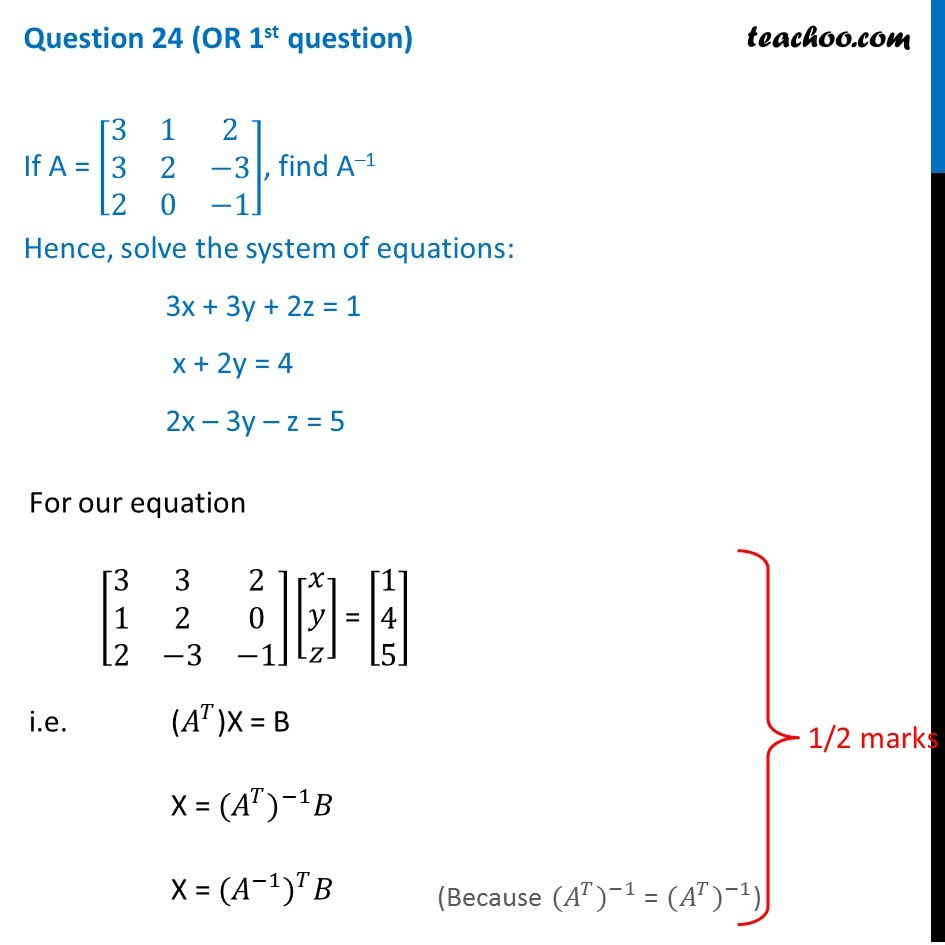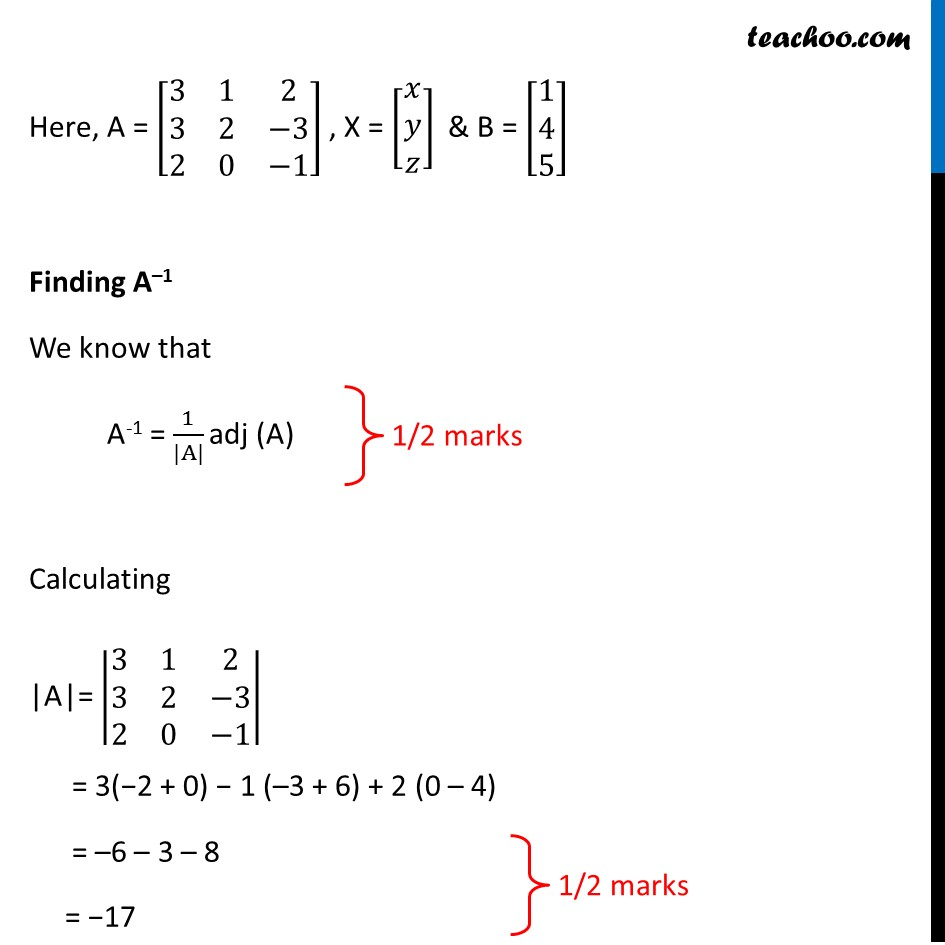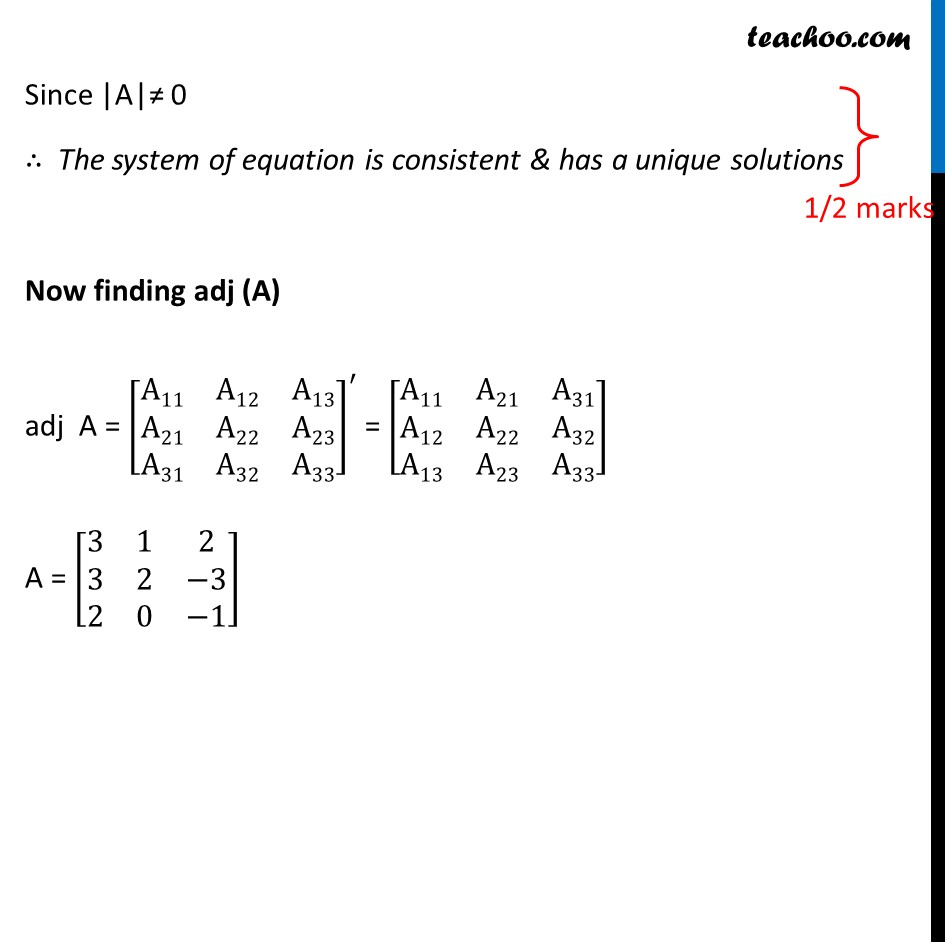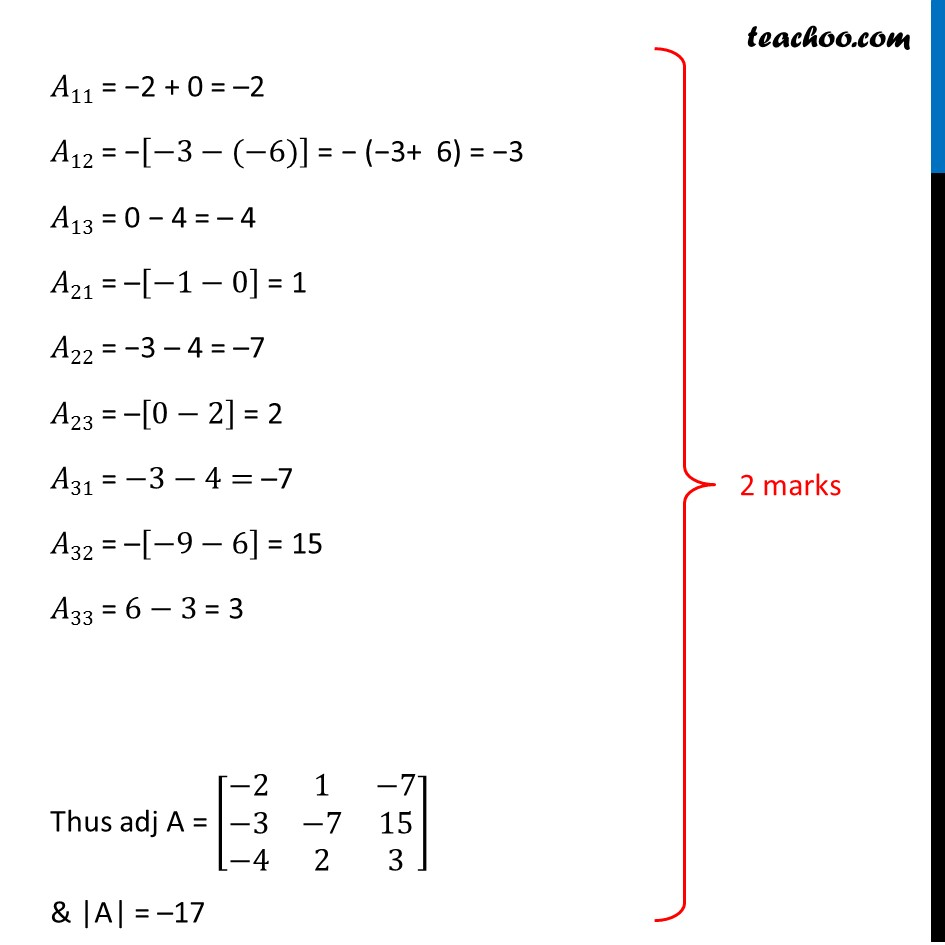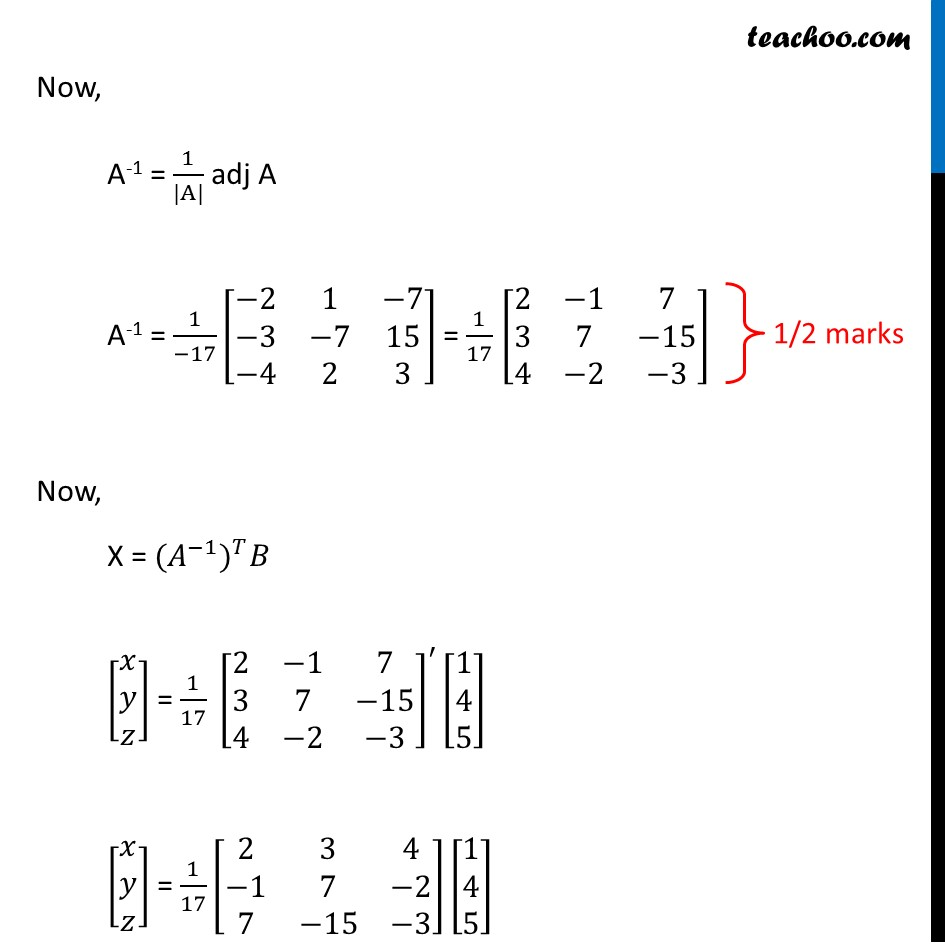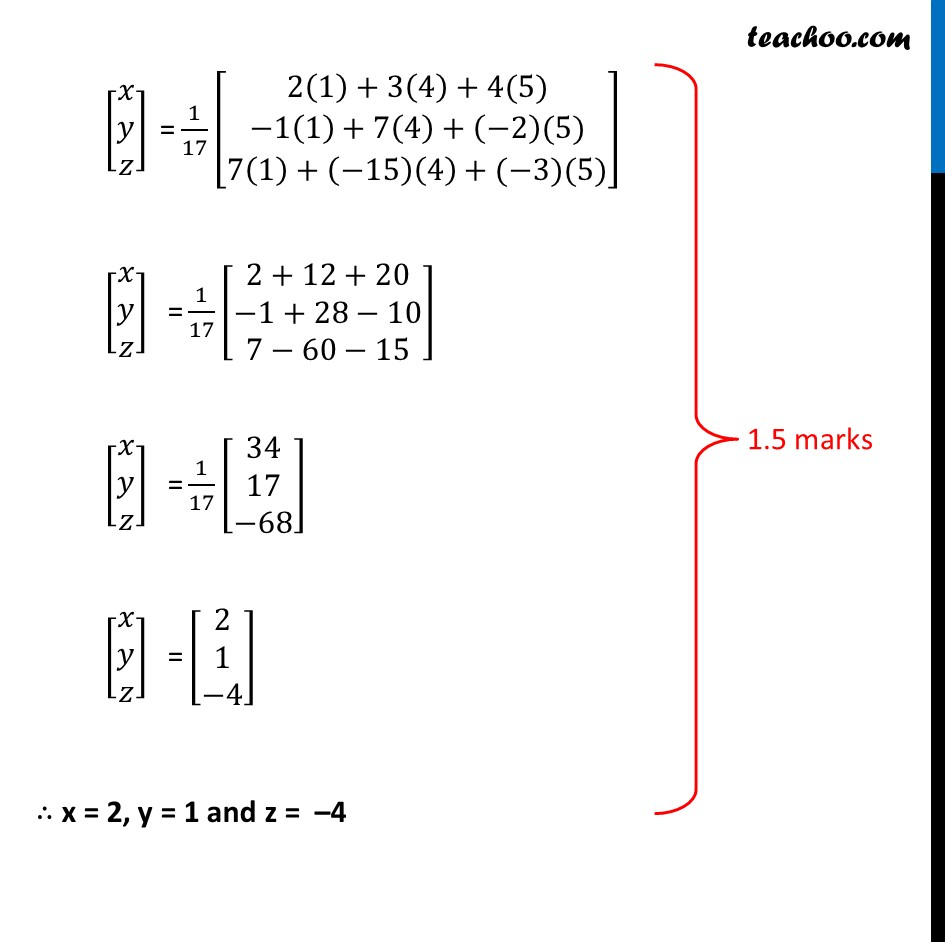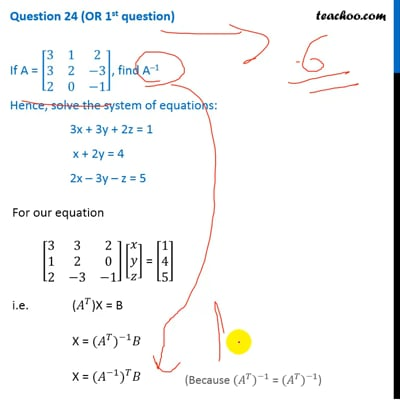This video is only available for Teachoo black users

Introducing your new favourite teacher - Teachoo Black, at only ₹83 per month

### Transcript

Question 24 (OR 1st question) If A = [■8(3&1&2@3&2&−3@2&0&−1)], find A–1 Hence, solve the system of equations: 3x + 3y + 2z = 1 x + 2y = 4 2x – 3y – z = 5 For our equation [■8(3&3&2@1&2&0@2&−3&−1)][■8(𝑥@𝑦@𝑧)] = [■8(1@4@5)] i.e. (𝐴^𝑇)X = B X = 〖(𝐴^𝑇)〗^(−1) 𝐵 X = 〖(𝐴^(−1))〗^𝑇 𝐵 (Because 〖(𝐴^𝑇)〗^(−1) = 〖(𝐴^𝑇)〗^(−1)) Here, A = [■8(3&1&2@3&2&−3@2&0&−1)] , X = [■8(𝑥@𝑦@𝑧)] & B = [■8(1@4@5)] Finding A–1 We know that A-1 = 1/(|A|) adj (A) Calculating |A|= |■8(3&1&2@3&2&−3@2&0&−1)| = 3(−2 + 0) − 1 (–3 + 6) + 2 (0 – 4) = –6 – 3 – 8 = −17 Since |A|≠ 0 ∴ The system of equation is consistent & has a unique solutions Now finding adj (A) adj A = [■8(A11&A12&A13@A21&A22&A23@A31&A32&A33)]^′ = [■8(A11&A21&A31@A12&A22&A32@A13&A23&A33)] A = [■8(3&1&2@3&2&−3@2&0&−1)] 𝐴11 = −2 + 0 = –2 𝐴12 = −[−3−(−6)] = − (−3+ 6) = −3 𝐴13 = 0 − 4 = – 4 𝐴21 = –[−1−0] = 1 𝐴22 = −3 – 4 = –7 𝐴23 = –[0−2] = 2 𝐴31 = −3−4= –7 𝐴32 = –[−9−6] = 15 𝐴33 = 6−3 = 3 Thus adj A = [■8(−2&1&−7@−3&−7&15@−4&2&3)] & |A| = –17 Now, A-1 = 1/(|A|) adj A A-1 = 1/(−17) [■8(−2&1&−7@−3&−7&15@−4&2&3)] = 1/17 [■8(2&−1&7@3&7&−15@4&−2&−3)] Now, X = 〖(𝐴^(−1))〗^𝑇 𝐵 [■8(𝑥@𝑦@𝑧)] = 1/17 [■8(2&−1&7@3&7&−15@4&−2&−3)]^′ [■8(1@4@5)] [■8(𝑥@𝑦@𝑧)] = 1/17 [■8(2&3&4@−1&7&−2@7&−15&−3)][■8(1@4@5)] " " [■8(𝑥@𝑦@𝑧)]" =" 1/17 [█(2(1)+3(4)+4(5)@−1(1)+7(4)+(−2)(5)@7(1)+(−15)(4)+(−3)(5))] " " [■8(𝑥@𝑦@𝑧)]" =" 1/17 [■8(2+12+20@−1+28−10@7−60−15)] " " [■8(𝑥@𝑦@𝑧)]" =" 1/17 [■8(34@17@−68)] " " [■8(𝑥@𝑦@𝑧)]" =" [■8(2@1@−4)] "∴ x = 2, y = 1 and z = "–4